×#### Thank you for registering.

One of our academic counsellors will contact you within 1 working day.

Click to Chat

1800-1023-196

+91-120-4616500

CART 0

• 0

MY CART (5)

Use Coupon: CART20 and get 20% off on all online Study Material

ITEM
DETAILS
MRP
DISCOUNT
FINAL PRICE
Total Price: Rs.

There are no items in this cart.
Continue Shopping• Complete JEE Main/Advanced Course and Test Series
• OFFERED PRICE: Rs. 15,900
• View Details

```Chapter 6: Algebraic Expressions and Identities Exercise – 6.4

Question: 1

Find the products

2a3(3a + 5b)

Solution:

To find the product, we will use distributive law as follows: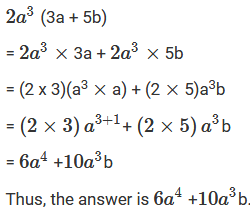Question: 2

Find the products

-11a (3a + 2b)

Solution:

To find the product, we will use distributive law as follows:.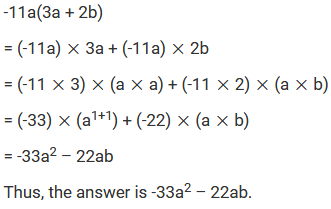Question: 3

Find the products

- 5a(7a – 2b)

Solution:

To find the product, we will use distributive law as follows: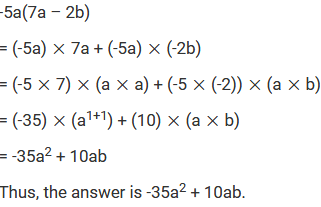Question: 4

Find the products

-11y2(3y + 7)

Solution:

To find the product, we will use distributive law as follows:Question: 5

Find the products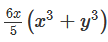Solution:

To find the product, we will use distributive law as follows:Question: 6

Find the products

xy (x3- y3)

Solution:

To find the product, we will use distributive law as follows: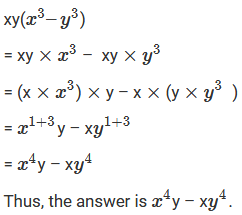Question: 7

Find the products

0.1y (0.1 x5 + 0.1y)

Solution:

To find the product, we will use distributive law as follows: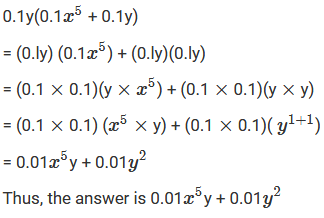Question: 8

Find the productsSolution:

To find the product, we will use distributive law as follows: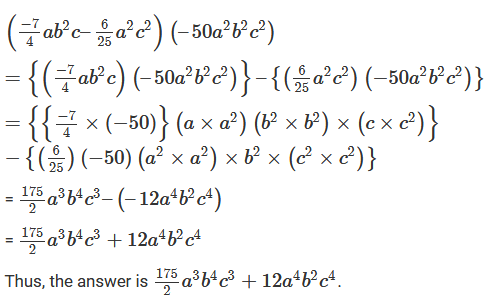Question: 9

Find the productsSolution:

To find the product, we will use distributive law as follows: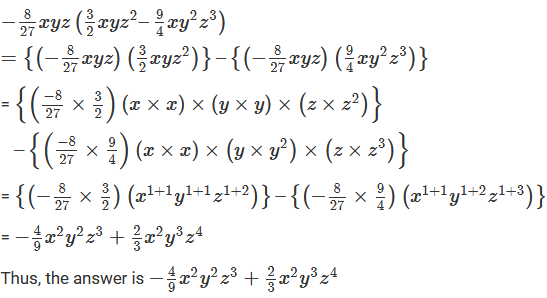Question: 10

Find the products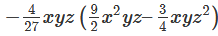Solution:

To find the product, we will use distributive law as follows: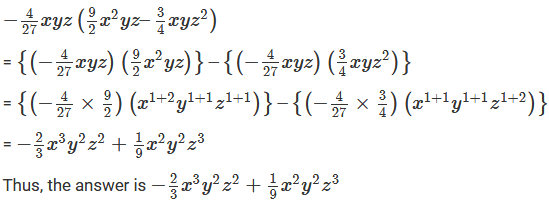Question: 11

Find the products

1.5 x(10x2y – 100xy2)

Solution:

To find the product, we will use distributive law as follows: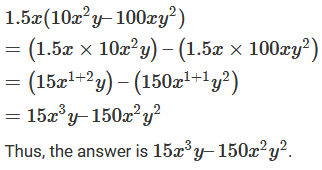Question: 12

Find the products

4.1 xy(1.1x – y)

Solution:

To find the product, we will use distributive law as follows:Question: 13

Find the products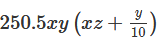Solution:

To find the product, we will use distributive law as follows: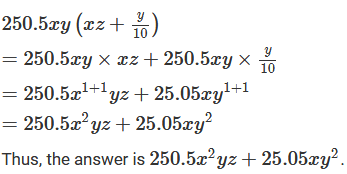Question: 14

Find the products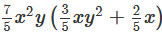Solution:

To find the product, we will use distributive law as follows: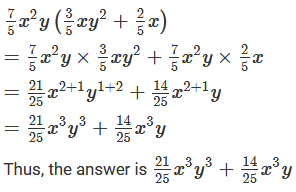Question: 15

Find the productsSolution:

To find the product, we will use distributive law as follows: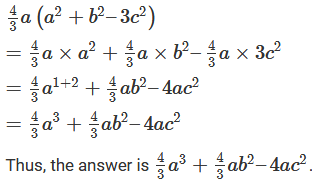Question: 16

Find the product 24x2(1-2x) and evaluate its value for x = 3.

Solution:

To find the product, we will use distributive law as follows: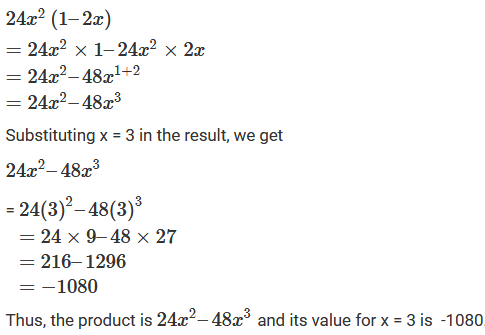Question: 17

Find the product -3y(xy + y2) and find its value for x = 4 and y = 5.

Solution:

To find the product, we will use distributive law as follows: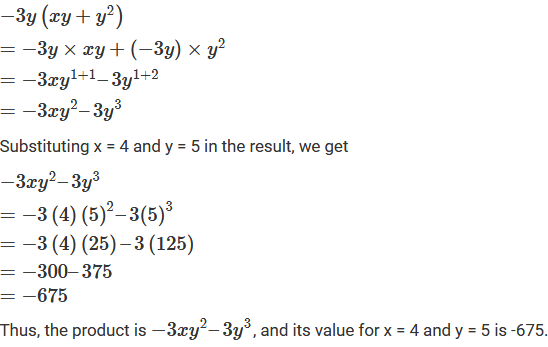Question: 18

Multiply – (3/2)x2y3 by (2x – y) and verify the answer for x = 1 and y = 2.

Solution:

To find the product, we will use distributive law as follows: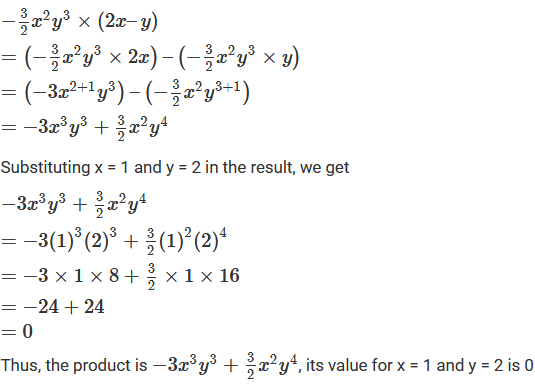Question: 19

Multiply the monomial by the binomial and find the value of each for x = -1, y = 0.25 and z = 0.05:

(i) 15y2 (2 – 3x)

(ii) −3x (y2 + z2)

(iii) Z2(x – y)

(iv) xz(x2 + y2)

Solution: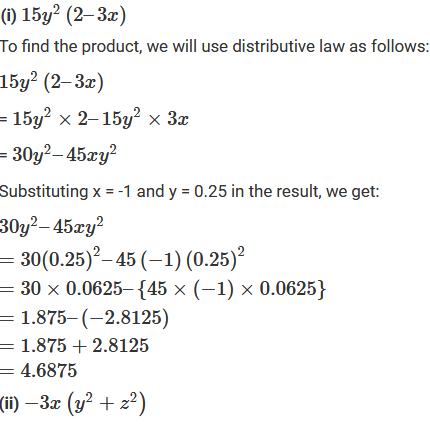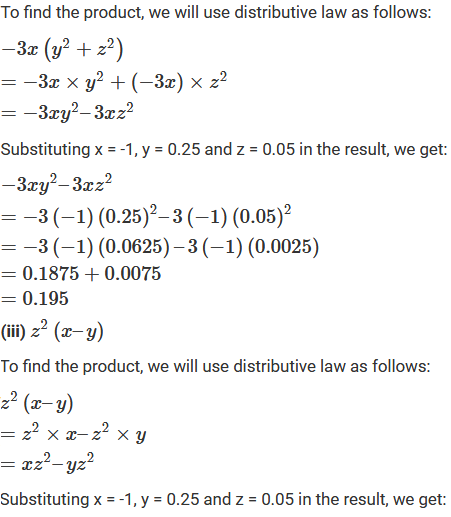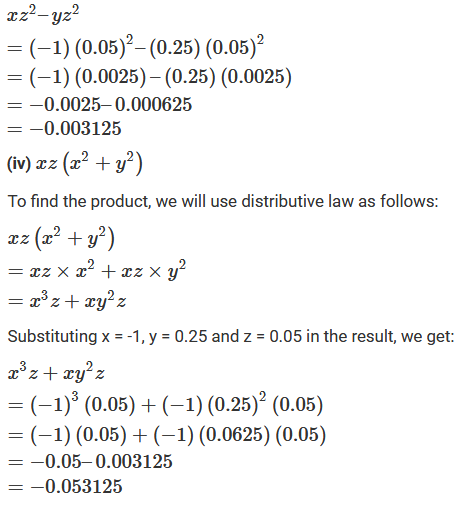Question: 20

Simplify:

(i) 2x2(x3 – x) –3x(x4+ 2x) -2(x4-3x2)

(ii) x3y (x2 – 2x) + 2xy(x3– x4)

(iii) 3a2 + 2(a + 2) -3a(2a + 1)

(iv) x(x + 4) + 3x(2x2–1) + 4x2 + 4

(v) a(b – c) – b(c – a) – c(a – b)

(vi) a(b – c) + b(c – a) + c(a – b)

(vii) 4ab(a–b) – 6a2(b – b2) –3b2(2a2 – a) + 2ab(b – a)

(viii) x2(x2 + 1) –x3(x + 1) –x(x3– x)

(ix) 2a2+ 3a(1- 2a3) + a(a + 1)

(x) a2(2a – 1) + 3a + a3 – 8(xii) a2b(a – b2) + ab2(4ab – 2a2) – a3b(1 – 2b)

(xiii) a2b(a3 – a + 1) – ab(a4 –2a2 + 2a) – b(a3 – a2 – 1)

Solution: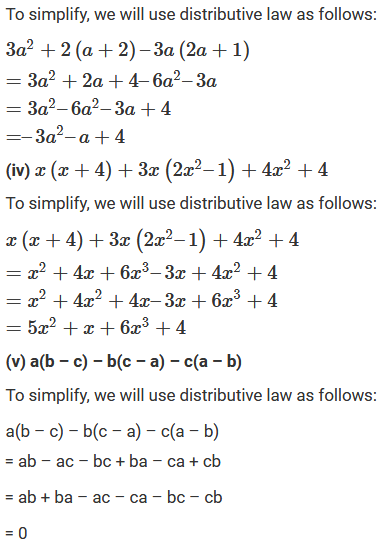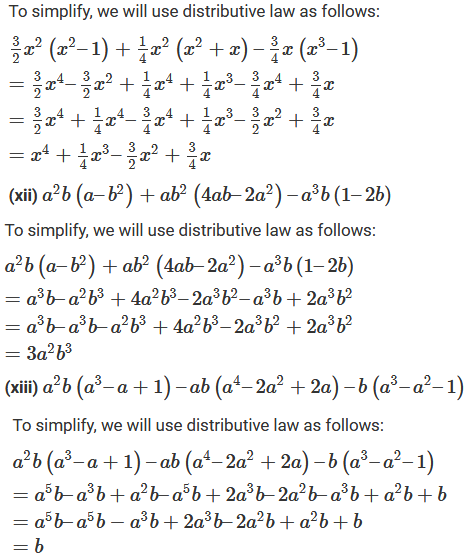```### Course Features

• 728 Video Lectures
• Revision Notes
• Previous Year Papers
• Mind Map
• Study Planner
• NCERT Solutions
• Discussion Forum
• Test paper with Video Solution